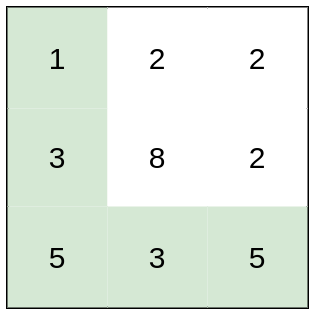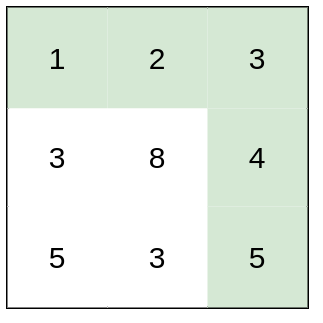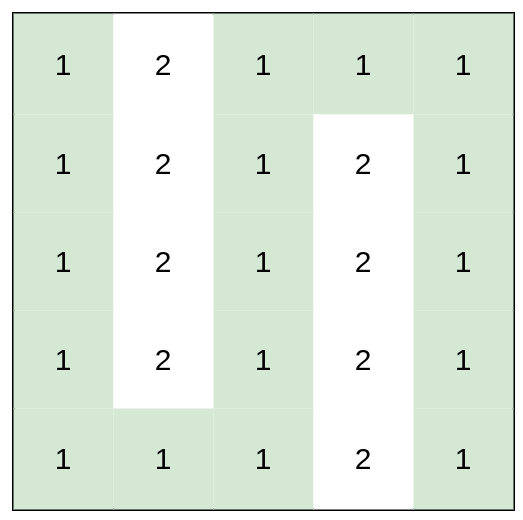Formatted question description: https://leetcode.ca/all/1631.html

# 1631. Path With Minimum Effort (Medium)

You are a hiker preparing for an upcoming hike. You are given heights, a 2D array of size rows x columns, where heights[row][col] represents the height of cell (row, col). You are situated in the top-left cell, (0, 0), and you hope to travel to the bottom-right cell, (rows-1, columns-1) (i.e., 0-indexed). You can move up, down, left, or right, and you wish to find a route that requires the minimum effort.

A route's effort is the maximum absolute difference in heights between two consecutive cells of the route.

Return the minimum effort required to travel from the top-left cell to the bottom-right cell.

Example 1:Input: heights = [[1,2,2],[3,8,2],[5,3,5]]
Output: 2
Explanation: The route of [1,3,5,3,5] has a maximum absolute difference of 2 in consecutive cells.
This is better than the route of [1,2,2,2,5], where the maximum absolute difference is 3.


Example 2:Input: heights = [[1,2,3],[3,8,4],[5,3,5]]
Output: 1
Explanation: The route of [1,2,3,4,5] has a maximum absolute difference of 1 in consecutive cells, which is better than route [1,3,5,3,5].


Example 3:Input: heights = [[1,2,1,1,1],[1,2,1,2,1],[1,2,1,2,1],[1,2,1,2,1],[1,1,1,2,1]]
Output: 0
Explanation: This route does not require any effort.


Constraints:

• rows == heights.length
• columns == heights[i].length
• 1 <= rows, columns <= 100
• 1 <= heights[i][j] <= 106

Related Topics: Binary Search, Depth-first Search, Union Find, Graph

Similar Questions:

## Solution 1. Dijkstra

// OJ: https://leetcode.com/problems/path-with-minimum-effort/

// Time: O(MNlog(MN))
// Space: O(MN)
class Solution {
public:
int minimumEffortPath(vector<vector<int>>& A) {
int M = A.size(), N = A.size(), dirs = { {0,1},{0,-1},{1,0},{-1,0} };
vector<vector<int>> dist(M, vector<int>(N, INT_MAX));
priority_queue<array<int, 3>, vector<array<int, 3>>, greater<>> pq;
dist = 0;
pq.push({ 0, 0, 0 });
while (pq.size()) {
auto [w, x, y] = pq.top();
pq.pop();
if (x == M - 1 && y == N - 1) return dist[x][y];
if (w > dist[x][y]) continue;
for (auto &[dx, dy] : dirs) {
int a = x + dx, b = y + dy;
if (a < 0 || b < 0 || a >= M || b >= N) continue;
int d = abs(A[x][y] - A[a][b]);
if (dist[a][b] > max(dist[x][y], d)) {
dist[a][b] = max(dist[x][y], d);
pq.push({ dist[a][b], a, b });
}
}
}
return -1;
}
};


Java

class Solution {
public int minimumEffortPath(int[][] heights) {
int rows = heights.length, columns = heights.length;
boolean[][] visited = new boolean[rows][columns];
int[][] values = new int[rows][columns];
int[][] directions = { {-1, 0}, {1, 0}, {0, -1}, {0, 1} };
PriorityQueue<int[]> priorityQueue = new PriorityQueue<int[]>(new Comparator<int[]>() {
public int compare(int[] array1, int[] array2) {
if (array1 != array2)
return array1 - array2;
else if (array1 != array2)
return array1 - array2;
else
return array1 - array2;
}
});
priorityQueue.offer(new int[]{0, 0, heights, values});
while (!priorityQueue.isEmpty()) {
int[] cell = priorityQueue.poll();
int row = cell, column = cell, height = cell, value = cell;
for (int i = 0; i < 4; i++) {
int[] direction = directions[i];
int newRow = row + direction, newColumn = column + direction;
if (newRow >= 0 && newRow < rows && newColumn >= 0 && newColumn < columns) {
if (!visited[newRow][newColumn]) {
visited[newRow][newColumn] = true;
values[newRow][newColumn] = Math.max(value, Math.abs(heights[newRow][newColumn] - height));
priorityQueue.offer(new int[]{newRow, newColumn, heights[newRow][newColumn], values[newRow][newColumn]});
} else {
int newValue = Math.max(value, Math.abs(heights[newRow][newColumn] - height));
if (newValue < values[newRow][newColumn]) {
values[newRow][newColumn] = Math.max(value, Math.abs(heights[newRow][newColumn] - height));
priorityQueue.offer(new int[]{newRow, newColumn, heights[newRow][newColumn], values[newRow][newColumn]});
}
}
}
}
}
return values[rows - 1][columns - 1];
}
}